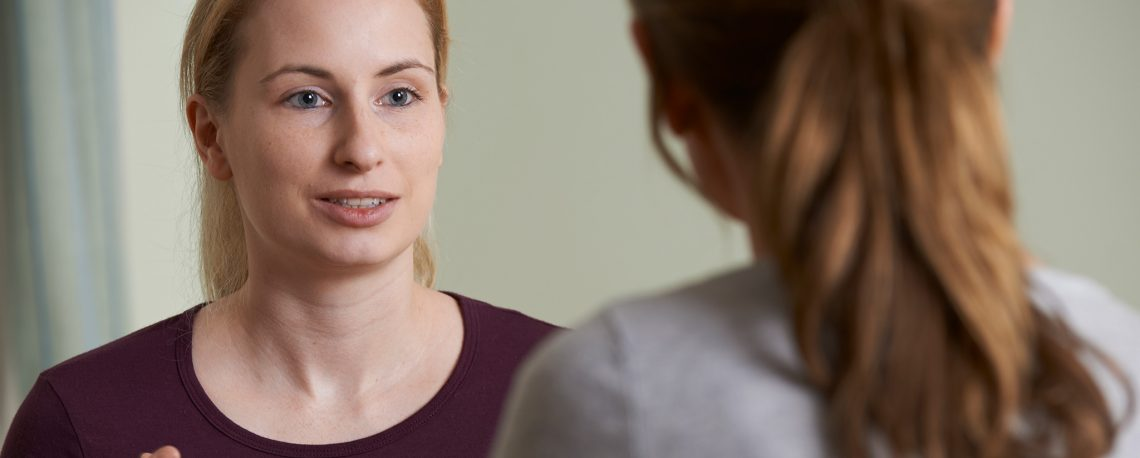# Here is an example article

This is where the body text of the article would go. This is where the body text of the article would go. This is where the body text of the article would go. This is where the body text of the article would go. This is where the body text of the article would go. This is where the body text of the article would go. This is where the body text of the article would go. This is where the body text of the article would go. This is where the body text of the article would go. This is where the body text of the article would go.

This is where the body text of the article would go. This is where the body text of the article would go. This is where the body text of the article would go.

This is where the body text of the article would go. This is where the body text of the article would go. This is where the body text of the article would go. This is where the body text of the article would go. This is where the body text of the article would go.